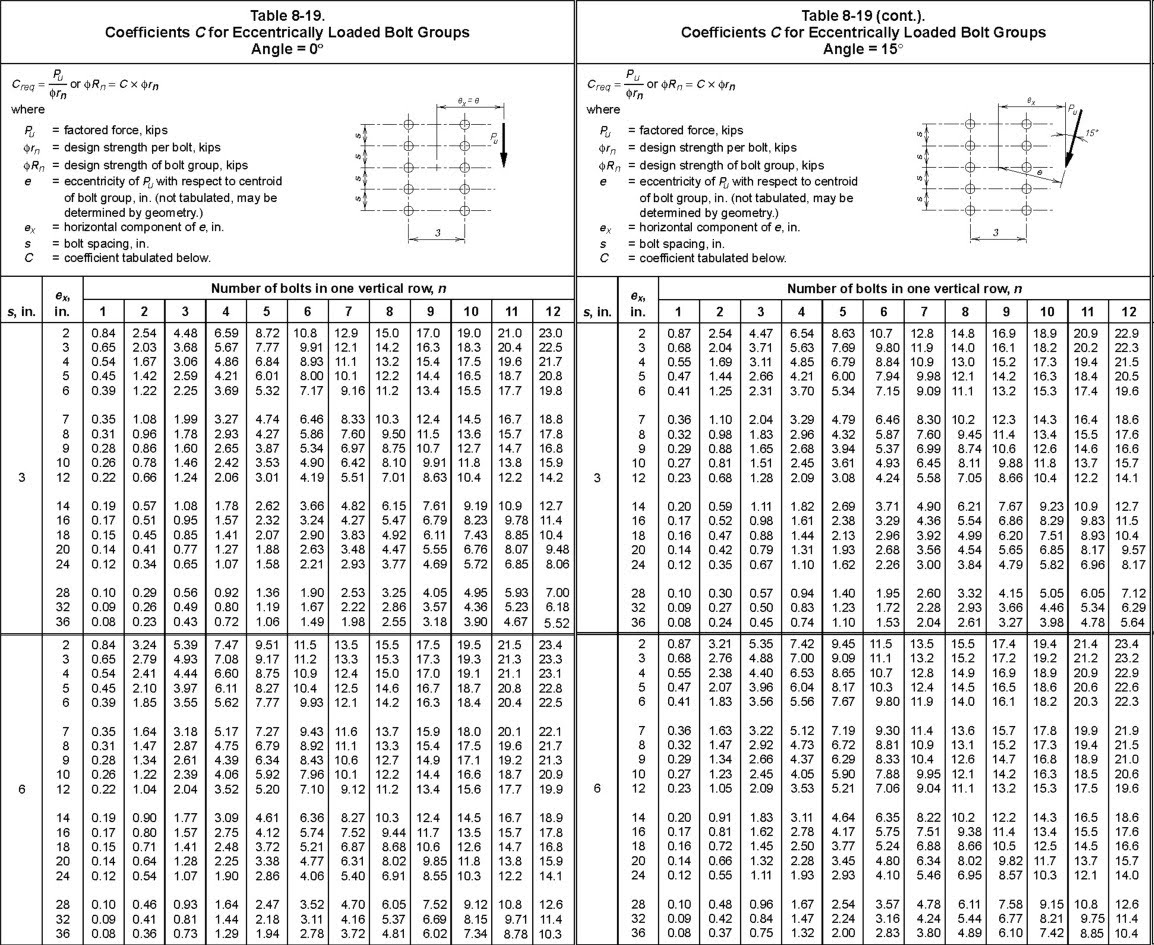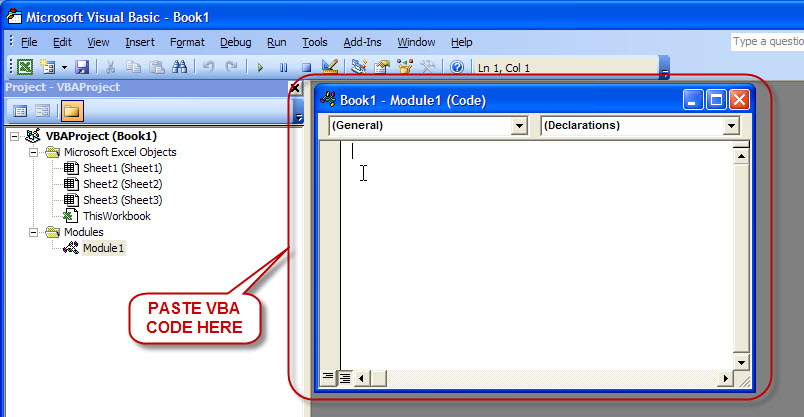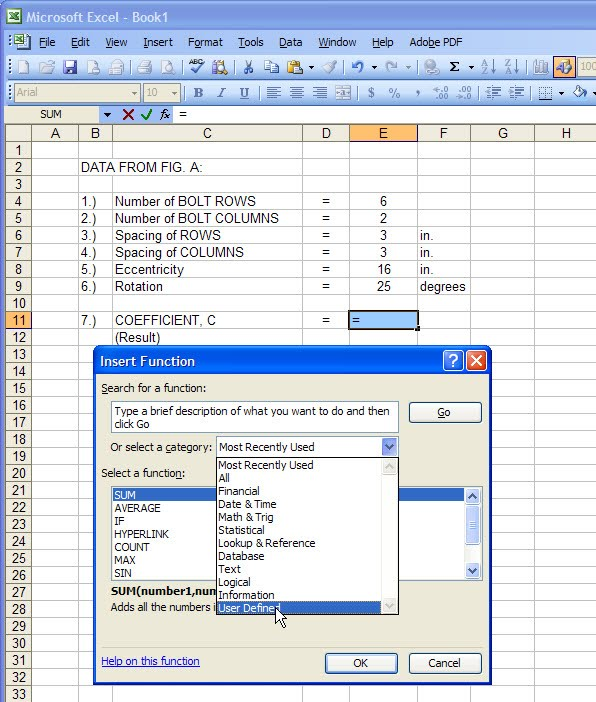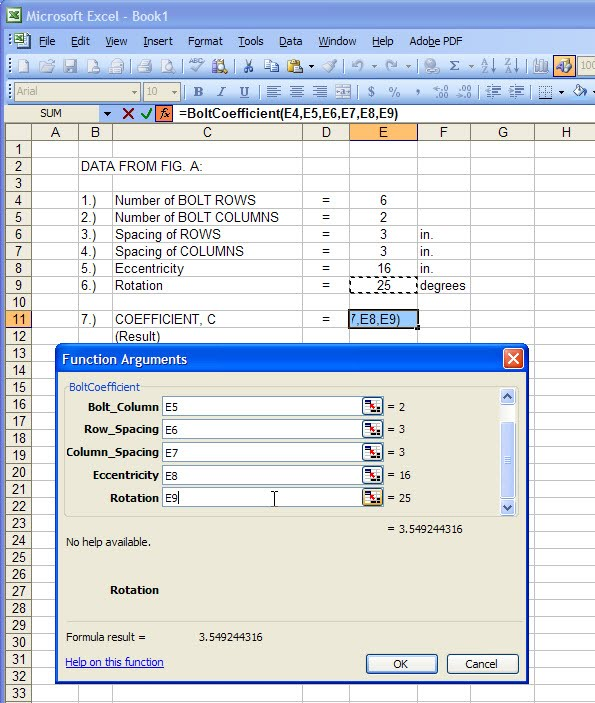# Eccentrically Loaded Bolt Group: Analysis and Design with VBA for Excel Implementation

0
127Once in a while I will be posting on this blog topics about engineering and structural design that are relevant to my job as structural designer. In this post I am going to share techniques in bolted connection calculation using MS Excel and VBA Codes (Visual Basic for Application).

There are two methods in the analysis of eccentrically loaded bolted connections presented by AISC Steel Construction Manual, namely:
1. The INSTANTANEOUS CENTER OF ROTATION Method; and
2. The ELASTIC Method.

These methods are discussed in AISC Steel Construction Manual and would be redundant if discussion is repeated here.In designing bolted connections, you may be confronted with both calculation ease and difficulties when you are employing the INSTANTANEOUS CENTER OF ROTATION Method (ICR). The Instantaneous Center of Rotation Method replaces the conservative but more popular ELASTIC Method which has a closed form analytical solution to the strength of the bolt group; meaning, it has an exact formula. The ICR, developed by Crawford and Kulak, is also known as ULTIMATE STRENGTH Method. Compared with the Elastic Method, ICR is found to be more economical since it is based on the ultimate strength of bolts. The only setback that the method has -- is that -- it requires intensive numeric iteration for a solution and cannot be performed by hand alone.

To preclude these difficulties, the AISC Steel Construction Manual devised a table to abbreviate the calculation of 'bolt group' strength. By providing the table, the formula for the strength of Bolt Group is simplified or reduced to Rn = C x rn; where Rn is the strength of bolt group, rn is the available strength of single bolt, and C is the coefficient provided for by the Table that corresponds to the effective number of bolts. With load eccentricity greater than zero, the coefficient C is always less than the total number of applied bolts. The reduction in the efficiency of bolt group is attributed to the increased load from the torque created by load eccentricity that tends to rotate the bolt group about the instantaneous center. Though the table makes the analysis and design of bolts much easier, it has apparent limitations which also make the design more difficult.

If you look at the tables for 'Coefficient C for eccentrically Loaded Bolt Groups' (pages 7-32 To 7-79 in AISC Steel Construction Manual 13th Edition) you will notice that the tables provided are valid only to loadings with angular positions of 0°, 15°, 30°, 45°, 60° and 75°. Other than these load angular positions, a cumbersome linear interpolation or extrapolation has to be performed to calculate the value of C. The bad thing is that the result of linear interpolation or extrapolation may not be correct: 'load eccentricity' to 'bolt group capacity' relation is not linear but a curve.

To use the table, let us consider calculating the capacity of the bolt group in Fig. A.Fig. A: Eccentrically Loaded Bolt Group

Assume a load P located 16" from the Center of Gravity (CG) of the bolt group. Also take note, by static equilibrium, load P corresponds to the capacity of bolts (or strength developed by bolt group) for the given eccentricity. By inspection, Table 8-19 (Angle = 0°) matches the bolt group configuration of Fig. A. The value for the coefficient C can then be derived readily from the table by locating on the horizontal row of the table the 'Number of bolts in one vertical Row, n' and then moving down along column 'ex' to locate the lookup value for eccentricity. With 6 vertical bolt rows and eccentricity of 16", the value of C is extrapolated from the table to be 3.24. Having determined the value of coefficient C, bolt capacity can then be calculated from the formula Rn = C x rn as discussed earlier.

The example above illustrates the ease in calculating capacity of bolt group with eccentric loading using tables. The drawback in this method, however, occurs when you have a bolt configuration whose eccentricity does not numerically concur with the lookup values on the table or when load angular position is not defined by any of the available bolt coefficient tables. Here are two scenarios:

1. When you have load eccentricity of 16.5” (using the same Fig. A), the value of C can not be readily extracted from the table. It is found somewhere between the values 3.24 and 2.9 that correspond to eccentricity lookup values 16” and 18”, respectively. An interpolation (which happen to be straight line in nature), therefore, is necessary.
2. When you have load with angular position other than 0°, 15°, 30°, 45°, 60° or 75°, there is no way one can solve the value of C using the tables. This is the problem shared by most engineers in the entire engineering community when designing bolted connections.

Fortunately, I have solved this problem long time ago by writing a program in Visual Basic that iterates and determines the correct location of the instantaneous center of rotation of a bolt group. With the IC located, the three equations of in-plane static equilibrium (ΣFx=0, ΣFy=0, ΣM=0) will be satisfied and capacity calculated. The program is based on the load-deformation relationship of bolt denoted by the equation,

R = Rult (1 – e-10Δ)0.55

where:

• R = nomimal shear strength of one bolt at a deformation Δ, in kips.
• Rult = ultimate shear strength of one bolt, kips
• Δ = total deformation, including shear, bearing and bending deformation in the bolt and bearing deformation of the connection elements, in.
• e = 2.718…, base of the natural logarithm.

Further discussion regarding this equation can be found on pages 7-6 to 7-8 of the AISC Steel Construction Manual, 13th Edition.

#### VBA Code: BOLT COEFFICIENT CALCULATOR

 1 2 3 4 5 6 7 8 9 10 11 12 13 14 15 16 17 18 19 20 21 22 23 24 25 26 27 28 29 30 31 32 33 34 35 36 37 38 39 40 41 42 43 44 45 46 47 48 49 50 51 52 53 54 55 56 57 58 59 60 61 62 63 64 65 66 67 68 69 70 71 72 73 74 75 76 77 78 79 80 81 82 83 84 85 86 87 88 89 `Option` `Explicit`   `Type BoltInfo` `   ``Dv ``As` `Double` `   ``Dh ``As` `Double` `End` `Type`   `'''Effective Bolt Coefficient` `Function` `BoltCoefficient(Bolt_Row ``As` `Integer``, Bolt_Column ``As` `Integer``, Row_Spacing ``As` `Double``, Column_Spacing ``As` `Double``, Eccentricity ``As` `Double``, ``Optional` `Rotation ``As` `Double` `= 0) ``As` `Double` `    ``Dim` `i, k, n ``As` `Integer` `    ``Dim` `mP, vP, Ro ``As` `Double` `    ``Dim` `Mo, Fy ``As` `Double` `    ``Dim` `xi, yi ``As` `Double` `    ``Dim` `Ri, x1 ``As` `Double` `    ``Dim` `y1, Rot ``As` `Double` `    ``Dim` `Rn, iRn ``As` `Double` `    ``Dim` `Rv, Rh ``As` `Integer` `    ``Dim` `Sh, Sv ``As` `Double` `    ``Dim` `Ec ``As` `Double` `    ``Dim` `Delta, Rmax ``As` `Double` `    ``Dim` `BoltLoc() ``As` `BoltInfo` `    ``Dim` `Stp ``As` `Boolean` `    ``Dim` `J ``As` `Double` `    ``Dim` `FACTOR ``As` `Double` `    `  `    ``Rv = Bolt_Row` `    ``Rh = Bolt_Column` `    ``Sv = Row_Spacing` `    ``Sh = Column_Spacing` `    `  `    ``ReDim` `BoltLoc(Rv * Rh - 1)` `    `  `    ``On` `Error` `Resume` `Next` `    `  `    ``Rot = Rotation * 3.14159265358979 / 180` `    ``Ec = Eccentricity * Cos(Rot)` `    `  `    ``If` `Ec = 0 ``Then` `GoTo` `ForcedExit` `    `  `    ``n = 0` `    ``For` `i = 0 ``To` `Rv - 1` `        ``For` `k = 0 ``To` `Rh - 1` `            ``y1 = (i * Sv) - (Rv - 1) * Sv / 2` `            ``x1 = (k * Sh) - (Rh - 1) * Sh / 2` `            ``With` `BoltLoc(n)` `                ``.Dv = x1 * Sin(Rot) + y1 * Cos(Rot) ``'''Rotate Vertical Coordinate` `                ``.Dh = x1 * Cos(Rot) - y1 * Sin(Rot) ``'''Rotate Horizontal Coordinate` `            ``End` `With` `            ``n = n + 1` `        ``Next` `    ``Next` `    `  `    ``Rn = 74 * (1 - Exp(-10 * 0.34)) ^ 0.55` `    ``Ro = 0: Stp = ``False` `    ``Do` `While` `Stp = ``False` `        ``Rmax = 0` `        ``For` `i = 0 ``To` `Rv * Rh - 1` `            ``xi = BoltLoc(i).Dh + Ro` `            ``yi = BoltLoc(i).Dv` `            ``Rmax = Application.WorksheetFunction.Max(Rmax, Sqr(xi ^ 2 + yi ^ 2))` `        ``Next` `        `  `        ``Mo = 0:  Fy = 0` `        ``mP = 0:  vP = 0` `        ``J = 0` `        ``For` `i = 0 ``To` `Rv * Rh - 1` `            ``xi = BoltLoc(i).Dh + Ro` `            ``yi = BoltLoc(i).Dv` `            `  `            ``Ri = Sqr(xi ^ 2 + yi ^ 2)` `            ``Delta = 0.34 * Ri / Rmax` `            ``iRn = 74 * (1 - Exp(-10 * Delta)) ^ 0.55` `            ``Mo = Mo + (iRn / Rn) * Ri                           ``'''Moment` `            ``Fy = Fy + (iRn / Rn) * Abs(xi / Ri) * Sgn(xi)       ``'''Vertical` `            ``J = J + Ri ^ 2` `        ``Next` `        ``mP = Mo / (Abs(Ec) + Ro)` `        ``vP = Fy` `        ``Stp = Abs(mP - vP) <= 0.0001` `        ``FACTOR = J / (Rv * Rh * Mo)` `        ``Ro = Ro + (mP - vP) * FACTOR   ` `        ``DoEvents` `    ``Loop` `    ``BoltCoefficient = (mP + vP) / 2` `    `  `    ``Exit` `Function` `ForcedExit:` `    ``BoltCoefficient = Rv * Rh` `End` `Function`
To implement the program to do your calculation, you need to copy and embed the VBA Code above to your excel spreadsheet. Here's how:

Step 1:

Open your excel spreadsheet where you intend to embed the VBA code. Here, for discussion purposes, let us use the data on Fig. A for a sample spreadsheet.

From the Tools menu under Macro as shown, click on Visual Basic Editor to activate the VBA Editor.Step 2:

As soon as the editor is activated, right click your mouse to display a pop-up menu.From the pop-up menu, choose Insert then click on Module. This will open a VBA module editor window as shown.On the VBA editor (assuming you have copied the VBA code above), paste the code.Once copied to the editor, the program is now ready for use.

Step 3:

The function BoltCoefficient defined in VBA code will now be recognized in Excel environment as a user defined function and can be called in any worksheet within the same workbook where the code is embedded. The function is called and applied in the same fashion as any other built-in math functions.
To test our BoltCoefficient function to calculate the value of C given the data from Fig. A, activate or select the cell where you intend to display or place the value; then click the fx button at the left side of the formula bar.A dialog box will pop-up showing available functions that you may want to use.The user defined function BoltCoefficient may not be found from the list of displayed functions. You may want to click on the category list box and click on User defined.The list of available User Defined function will be displayed. Select and click on BoltCoefficient then click on OK button.The Function Arguments dialog box is displayed showing the function BoltCoefficient arguments that need to be supplied to calculate C. Input box is made available at the right side of argument description. You can specify or supply the arguments by directly typing the value onto the box or by specifying the cell address where the value is located. In our example, I opted to specify the arguments with the address of each cell. Notice that at the right side of the box, the value of cell is also displayed. Also notice that as soon as you supplied all the necessary arguments, the function automatically returns the value 3.549244316. This is the value of the Bolt Coefficient C!

The calculated value of C is the result from the following inputs:

1. Number of Bolt Rows = 6
2. Number of Bolt Columns = 2
3. Spacing of Rows = 3
4. Spacing of Columns = 3
5. Eccentricity = 16